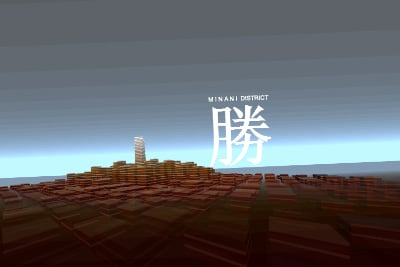# MINAMI DISTRICT

567 words ~ 3-5 minsMathieu 'p01' Henri on June 29th, 2013

# Minami District

DEMOJS 2013's winner 1k intro. With MINAMI DISTRICT I wanted to do something fresh. Something never seen in normal demos before: A city with a twister skyscraper.There is also an archive released at DEMOJS 2013 including the packed and unpacked versions.

## FILE_ID.DIZ

``````   1,024 bytes self extracting png.html
1 imaginary epic music
1 3D engine
793 translucent buildings
24,257 polygons with haze``````

## Planning and ploting

I expected the competition to ramp up after MATRAKA which took 4 weeks to produce, so there was no other choice but to work hard and use the whole three months between JS1k 2013 and DEMOJS 2013.

The idea of the twisting skyscraper came very early. But a skyscraper alone isn't really interesting, it needs a city around it to twist and shine.

So I spent most of these three months trying dozens of techniques to render and twist buildings in 3D. The only thing I did not try was webGL, because from experience it was too verbose for this project.

## You name it

Rendering and animating 1024 buidings in 1024 bytes is not trivial.

One of the first idea was to go full on with particles, but it took up to 40.000 particles to make up one building. This would allow t blow things up and transform things at will, but that didn't scale one bit.

Next was to render on segment per floor/window, stretching the particles if you will. It reduced the number of elements dramatically but had annoying artefacts at the ends of the segments.

Then came the CSS3D experiments. Sure enough the FAMO.US tech demo looked impressive and smooth, but it never featured more than 200 elements. That is exactly the tipping point where performance collapsed in my own tests. Again, this was not an option.

Eventually I managed to render each wall and window of each floor of the buildings as polygons. In hindsight, this was the only realistic option. Although I would give webGL a go.

## Sorting

The buildings are sorted and drawn back to front, using their center, then the walls of each floor are drawn bottom up, going clock wise around the building and comparing the x coordinate of each edge of the wall or window to determine whether it is facing the camera or not. This sorting was quite compact and works in most cases.

## Silence

To much chagrin, I had to sacrifice the music. The visuals were catchy enough to get away without sound this one time. But I promise I will try hard to not repeat this.

## Source code

The source code below assumes the following setup which comes from the PNG bootstrapping

``<canvas id=c><script>a=c.getContext('2d');S=String.fromCharCode;</script>``
``T=[Q=Math.cos,c.style.cssText="position:fixed;top:0;left:0;width:100%;height:100%",A=Date.now()/1024];setInterval(function u(v,w,x,y){if(v){if(x){a.beginPath();a.moveTo(v.x,v.y),a.lineTo(w.x,w.y),(x.x?(a.lineTo(x.x,x.y),a.lineTo(y.x,y.y)):(a.lineTo(w.x,w.y+x*w.p),a.lineTo(v.x,v.y+x*v.p),a.fillStyle=c[y]));a.fill()}return w.z-v.z}c.width=w=768;c.height=h=w*innerHeight/innerWidth|0;o=h*2/3;O=Date.now()/1024-A;e=O/12;Z=128*Q(e/2+11)-256;T.sort(u);a.rotate((O/17&13)/32-.2);v={x:-w,y:o,p:1};s={x:w*2,y:o,p:1};for(i=0;i<32;i++){y=Math.max(0,h*256/(Z+i*128));u(v,s,y/2,i*8+2);u(v,s,-y,i*8+3);}a.fillStyle=c[w];a.fillText("M I N A M I  DISTRICT",w/2+8,o-128);a.font="bold 128px a";a.fillText(S(21213),w/2,o-16);for(i=0;i<1024;i++){k=i/2&3;j=(i/4&-1)+(i&1)*32;M=j-k*16+32*Q(e)|0;j=j-k*64*Q(e)|0;c[i]="rgb("+[j+=M,j+=M,j+=M]+")";v=T[i];if(v&&v.z>16&&v.k<256){y=-16*Q(e/4)-24;s=v.k?8-2*Q(v.i):11;H=v.k?Math.max(2,11-v.k/8-4*Q(s-v.k))+(s-v.k&1):32;k=i;for(j=0;j<H;j++){y+=4;a.globalAlpha=1-v.k/(128+j*64);C=Q(e);D=Q(e+11);for(i=0;i<5;i++){x=i&2?s:-s;z=1+i&2?s:-s;p=256/(v.z+z*C-x*D);T[i]={p:p,x:w/3+p*(v.x+z*D+x*C),y:o-p*y};if(i&&T[i].x<t.x){u(T[i],t,4,j*8+i*2);u(T[i],t,1,j*8+i*2+1);}t=T[i]}e+=v.k?0:Q(Math.max(0,O/17-1)+11)/6}i=k;e=O/12;if(y<0)u.apply(T,T)}C=Q(e);D=Q(e+11);x=(i&31)-16;z=(i>>5)-16;T[i]={i:i,k:x*x+z*z,z:24*(z*C-x*D)-Z,x:24*(z*D+x*C)}}},1)``